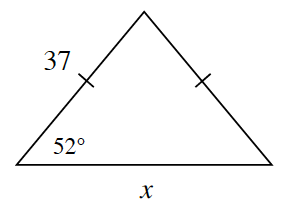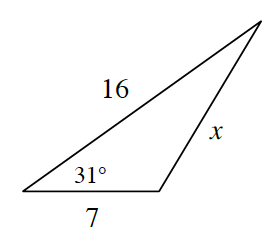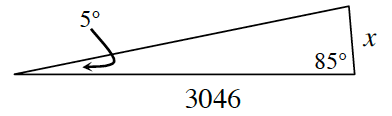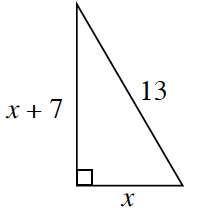Home > CCG > Chapter 6 > Lesson 6.2.1 > Problem6-55

6-55.

Use your triangle tools to solve for $x$ in the triangles below.

1.What do the tick marks on the sides of the triangle mean?
What do they tell you about the lengths of the sides and the measures of the angles?

Use the Law of Sines to find $x$.

$x\approx45.56$

1.Use the Law of Cosines to solve for $x$.

$x\approx10.63$

1.Find the measure of the missing angle.

Redraw the triangle with the angles to scale.

$x\approx265.48$

1.What special right triangle has a hypotenuse of $13$?